# Electronics and Communication Engineering - Exam Questions Papers

16.

(11)2 + (11)3 = (11)?

 A. 4 B. 5 C. 6 D. none

Explanation:

(10)2 + (11)3 = (11)7

⇒ 2 + 1 + 3 + 1 = x + l ⇒ x = 6.

17.

For a given transfer function: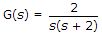Damping ratio is:

 A. 0.707 B. 0.695 C. 1.414 D. 0.798

Explanation: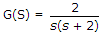1 + G(s)H(s) = s2 + 2s + 2

ωn = 2 , 2εeωn = 2, εe =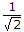= 0.707.

18.

The energy of constant-amplitude complex valued exponential sequence is __________ (where A is the constant amplitude)

 A. 0 B. 1 C. ∞ D. A2

Explanation:

Let the constant amplitude complex valued exponential sequence.

x(n) = Ae-jωn

Energy =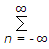|x(n)|2 =|Ae-jωn|2

A2|(e-jωn)|2 = A2(∞) = ∞

Hence energy in infinite.

19.

Find the electric charge required on the earth and moon to balance their gravitational attraction if the charge on the earth is 10 times that on the moon.

 A. 1.73 TC B. 17.3 GC C. 17.3 TC D. 1.73 GC

Explanation:

Using law of gravitations, the force between the two point masses is F =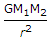M1 = mass of moon = 6.7 x 1022 Kg

M2 = mass of earth = 6 x 1024 Kg

r = distance between masses = 380 mm

G = universal gravitational constant = 6.7 x 10-11 Nm2/Kg2

Let Q1 and Q2 be the charge on moon and earth respectively. Now the gravitational force must be balanced by the force of repulsion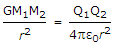Q1Q2 = 4pε0M1M2G

but Q2 = 10Q1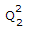= 10 x 4pω0M1M2G

Q2 = 173 x 1012

Q1 = 17.3 x 1012 C

Q1 = 17.3 TC.

20.

An audio signal of FM radio station is sampled at a rate of 15% above the Nyquist rate. Signal is sampled in 512 levels and bandwidth of the signal is 4 KHz. The minimum bandwidth required to transmit this signal will be

 A. 77 K bits/sec B. 92 K bits/sec C. 84.8 K bits/sec D. 82.8 K bits/sec

Explanation:

Nyquist rate = 2 x 4 kHz = 8 kHz

Sampling rate = 8 kHz + 1.2 kHz = 9.2 kHz

Number of bits required = log2512 = 9

Thus bandwidth = 9 x 9.2 = 82.8 k bits/sec.

#### Current Affairs 2022

Interview Questions and Answers